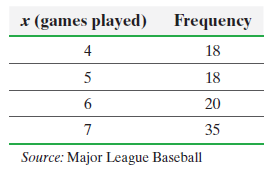×
Get Full Access to Statistics: Informed Decisions Using Data - 5 Edition - Chapter 6.1 - Problem 21
Get Full Access to Statistics: Informed Decisions Using Data - 5 Edition - Chapter 6.1 - Problem 21

×

# ?In Problems 21 and 22, (a) construct a discrete probability distribution for the random variable X (b) draw a graph of the probability distribution,ISBN: 9780134133539 240

## Solution for problem 21 Chapter 6.1

Statistics: Informed Decisions Using Data | 5th Edition

• Textbook Solutions
• 2901 Step-by-step solutions solved by professors and subject experts
• Get 24/7 help from StudySoup virtual teaching assistantsStatistics: Informed Decisions Using Data | 5th Edition

4 5 1 369 Reviews
18
2
Problem 21

In Problems 21 and 22, (a) construct a discrete probability distribution for the random variable X(b) draw a graph of the probability distribution, (c) compute and interpret the mean of the random variable X, and (d) compute the standard deviation of the random variable X.

The World Series The following data represent the number of games played in each World Series from 1923 to 2014.Step-by-Step Solution:

Step 1 of 5) Draw a graph of the probability distribution, (c) compute and interpret the mean of the random variable X, and (d) compute the standard deviation of the random variable X. The World Series The following data represent the number of games played in each World Series from 1923 to 2014. If a data value is larger than the mean, the z-score is positive. If a data value is smaller than the mean, the z-score is negative. If the data value equals the mean, the z-score is zero. A z-score measures the number of standard deviations an observation is above or below the mean. For example, a z-score of 1.24 means the data value is 1.24 standard deviations above the mean. A z-score of -2.31 means the data value is 2.31 standard deviations below the mean. In Other Words The z-score provides a way to compare apples to oranges by converting variables with different centers or spreads to variables with the same center (0) and spread (1).

Step 2 of 2

## Discover and learn what students are asking

Statistics: Informed Decisions Using Data : Testing the Significance of the Least-Squares Regression Model
?True or False: In a least-squares regression, the response variable is normally distributed with mean my!x and standard deviation s.

Unlock Textbook Solution